# How to Convert Date to Day of Week in Excel (3 Easy Ways)

When working with date-specific information, there might be instances where you need to find the day of the week corresponding to a date.

For example, instead of the date, you may want to know whether it’s a Monday or a Tuesday or any other day.

This could be useful when working with to-do lists, daily/weekly summary reports, etc.

In this tutorial, we will see three different ways of converting dates to the corresponding day of the week in Excel.

For all the three methods in this tutorial, we will be using the following sample dataset.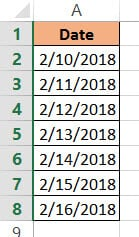We will convert all the dates in the above sample dataset into corresponding days of the week.

## Method 1: Converting Date to Day of Week in Excel using the TEXT Function

The TEXT function is a great function to convert dates to different text formats. It takes a date serial number or date and lets you extract parts of the date to fit your required format.

Here’s the syntax for the TEXT function:

`= TEXT (date, date_format_code)`

In this function,

• date is the date, date serial number, or reference to the cell that you want to convert.
• date_format_code is the format code based on how you want the converted date to look.

The TEXT function applies the date_format_code that you specified on the provided date and returns a text string with that format.

For example, if you have the date “2/10/2018” in cell A2, then =TEXT(A2,”dddd”) will return “Saturday”. Here, “dddd” is the date_format code to display the day of the week corresponding to date in full words.

Here are some basic building blocks for the format codes that you can use:

### Format Codes for Day of the Week or Month:

You can use the following basic format codes to represent day values:

• d – one or two-digit representation of the day (eg: 3 or 30)
• dd – two-digit representation of the day (eg: 03 or 30)
• ddd – the day of the week in abbreviated form (eg: Sun, Mon)
• dddd – full name of the day of the week (eg: Sunday, Monday)

So,

• if you apply =TEXT(A2, “d”) to the sample dataset, it will return “10”.
• if you apply =TEXT(A2, “dd”), then it will return “10”.
• if you apply =TEXT(A2, “ddd”) to the sample dataset, it will return “Sat”.
• if you apply =TEXT(A2, “dddd”), then it will return “Saturday”.

Let us see how we can apply the TEXT function to our sample dataset to convert all the dates to days of the week.

We will first see how to convert the dates in column A to the format shown in column B in the image below: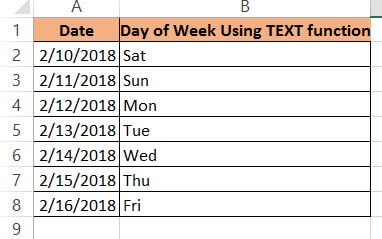Below are the steps to convert date to weekday name using the TEXT function:

1. Click on a blank cell where you want the day of the week to be displayed (B2)
2. Type the formula: =TEXT(A2,”ddd”) if you want the shortened version of the day or =TEXT(A2,”dddd”) if you want the full version of the days.
3. Press the Return key.
4. This should display the day of the week in our required format. Copy this to the rest of the cells in the column by dragging down the fill handle or double-clicking on it.
5. Copy this column’s formula results by pressing CTRL+C or Cmd+C (if you’re on a Mac).
6. Right-click on the column and from the popup menu that appears, press Paste Values from the Paste Options.
7. This will store the formula results as permanent values in the same column. Now you can go ahead and remove column A if you want to.

You should see all the dates converted to the short form of their corresponding days of the week: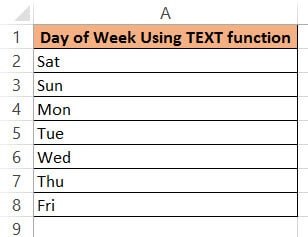The result you get in column B will differ according to the format code you used in step 2. Here are some format codes along with the type of result you will get when applied to cell A2:

 Function Result =TEXT(A2, “ddd”) Sat =TEXT(A2, “dddd”) Saturday

Note: Using this formula, your converted date is in text format, so you will see the results aligned to the left side of the cells.

## Method 2: Converting Date to Day of Week in Excel using the Format Cells Feature

The Format Cells feature provides a great way to directly convert your dates to days of the week while replacing the existing dates. As such you don’t need to have a separate column to type the formula.

You can use the Format Cells dialog box to convert your dates to days of the week as follows:

1. Select all the cells containing the dates that you want to convert (A2:A8).
2. Right-click on your selection and select Format Cells from the popup menu that appears. Alternatively, you can select the dialog box launcher in the Number group under the Home tab.
3. This will open the Format Cells dialog box. Click on the Number tab
4. Under Category on the left side of the box, select the Custom option.
5. This will display a number of formatting options on the right side, under Type. You can type in your format code in the Type field. You can type in “ddd” if you want the short form or “dddd” if you want the full form of the day.
6. Click OK to close the Format Cells dialog box.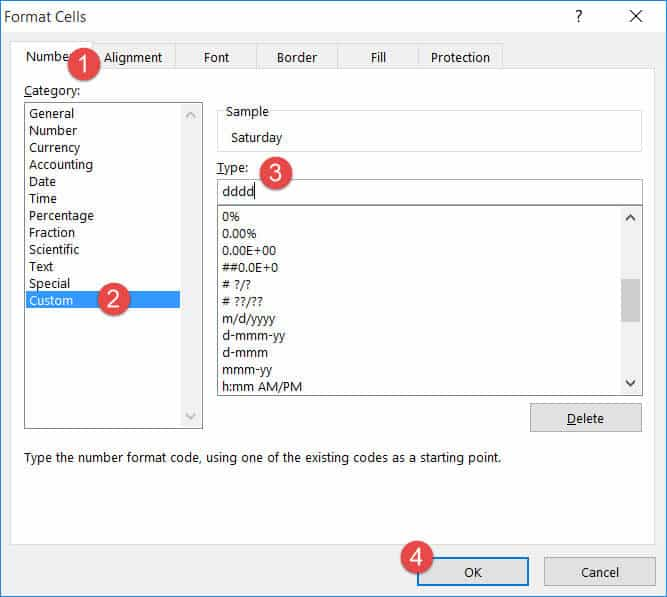All your selected cells should now appear as weekday names.

Note: By using Format Cells, you have just changed the format in which the dates are displayed in the cells. The actual dates are underlying the cells and can be seen in the formula bar when a cell is selected.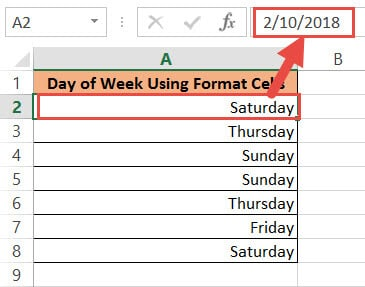## Method 3: Converting Date to Day of Week in Excel using a Formula

If you’d rather use your own representation for the days of the week, you can use a formula based on both the WEEKDAY and CHOOSE functions.

First, let us understand these two functions individually.

### The WEEKDAY Function

The WEEKDAY function converts a date to a number between 1 and 7, one for each day of the week. It chooses the sequencing of these numbers based on the day we want the week to begin from.

This is helpful because in some countries, the week starts from Sunday, while in others, especially Middle Eastern countries, the week starts from Saturday.

The syntax for the WEEKDAY function is:

WEEKDAY(date, [type])

Here,

• date is the input date for the function. It can be a date, a date serial number or a reference to a cell that holds a date value.
• type is an optional parameter. It tells the function where to begin the week from. So, if we put a 1, it means the week should start from Sunday and end on Saturday. If we put a 2, it means the week should start from Monday and end on Sunday, and so on. By default, the value for the type parameter is set to 1.

In our example, WEEKDAY(A2, 1) will return 7, corresponding to a Saturday.

### The CHOOSE Function

The CHOOSE function lets you specify a particular outcome depending on a value. For example, you can choose to display the word “red” if a value is 1, “blue” if it is 2, “green” if it is 3, and so on.

In this way it’s a great alternative to using a bunch of nested IF functions.

The syntax for the CHOOSE function is:

=CHOOSE (index, value1, [value2], …)

Here,

• index is the input value. It can be any number between 1 and 254.
• value1 is the first value from the list of possible outputs
• value2 is the second value from the list of possible outputs,

etc.

The function returns value1 if index is 1, value2 if index is 2, etc.

So the function: =CHOOSE(3, “sun”,”mon”,”tue”,”wed”,”thu”,”fri”, ”sat”) will return “tue”, because it’s the third value in the list.

### Putting the Formula Together

Let us put the two functions CHOOSE and WEEKDAY together to convert date to day of the week. Here are the steps to follow:

1. Click on a blank cell where you want the day of the week to be displayed (B2)
2. Type the formula: =CHOOSE(WEEKDAY(A2),”Sun”,”Mon”,”Tue”,”Wed”,”Thu”,”Fri”,”Sat”)
3. Press the Return key.
4. This should display the day of the week in short form, corresponding to the date in A2. Copy this to the rest of the cells in the column by dragging down the fill handle or double-clicking on it.
5. Copy this column’s formula results by pressing CTRL+C or Cmd+C (if you’re on a Mac).
6. Right-click on the column and form the popup menu that appears, press Paste Values from the Paste Options.
7. This will store the formula results as permanent values in the same column. Now you can go ahead and remove column A if you want to.

Here’s what you should get as the result: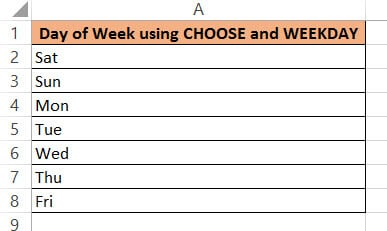#### How did this Formula Work?

To understand how this formula worked, we need to break it down:

1. First, we used the WEEKDAY function to find the number corresponding to the day of the week, assuming our week starts from Sunday. This will return a number between 1 and 7.
2. We used the output of the WEEKDAY function to find the string from the list of days [“sun”,”mon”,”tue”,”wed”,”thu”,”fri”, “sat”] corresponding to that number. So if the output for WEEKDAY(A2) was 7, then the corresponding output for the CHOOSE function will be the seventh item in the list, which is “sat”.

Thus we get the day corresponding to the date in A2 (in our example) to be “sat”.

Note: The above formula will give your converted date in text format, so you will see the results aligned to the left side of the cells.

You could also have any other format for the days in the list, for example, if you wanted to display just the first two letters for the days, you could have written the formula in step 2 as:

`=CHOOSE(WEEKDAY(A2),"Su","Mo","Tu","We","Th","Fr","Sa")`

So you could get an output as: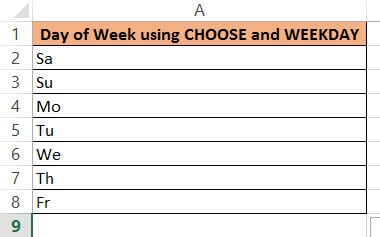If you wanted to make a to-do list, you could even have an activity for each day of the week. For example, you could use the formula:

`=CHOOSE(WEEKDAY(A2),"cycling","rollerblading","swimming","skiing","art","crafts","free time")`

So you could get an output as: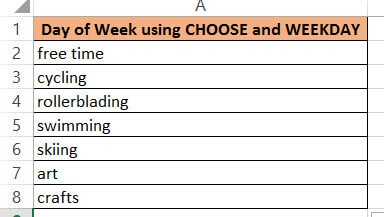In this tutorial, we saw three ways to convert a date to the day of the week.

The first method involves a function (TEXT), the second method involves a commonly used Excel dialog box (Format Cells) and the third method involves an Excel formula (with WEEKDAY and CHOOSE functions).

We hope you found this tutorial helpful and easy to apply to your data.

Other Excel tutorials you may find useful: